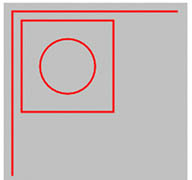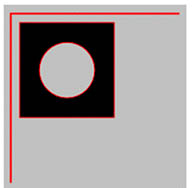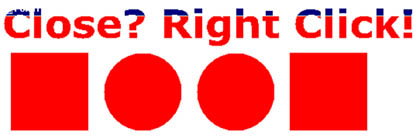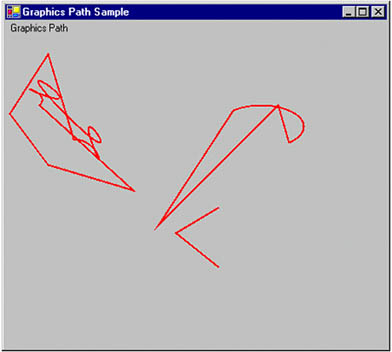# Understanding and Using Graphics Paths

In Chapter 3 we briefly discussed how to create a graphics path, add graphics items to the path, and draw and fill graphics paths using FillPath and DrawPath.

A graphics path is a set of connected lines, curves, and other simple graphics objects, including rectangles, ellipses, and text. A path works as a single graphics object, so an effect applied to the graphics path will be applied to all the components of the path. For example, if a graphics path contains a line, a rectangle, and an ellipse and we draw the path using a red pen, all three components (line, rectangle, and ellipse) of the graphics path will be drawn with the red pen.

To create and use a graphics path, we create a GraphicsPath object and add its components by using add methods. For example, you can use the AddLine, AddRectangle, and AddEllipse methods to add a line, a rectangle, and an ellipse, respectively, to the graphics path. After adding components to a path, you can use DrawPath or FillPath to draw and fill it.

By default, all graphics shapes of a path are connected to one another and treated as a single entity with a collection of points and point types. But by using StartFigure and CloseFigure, an application can draw more than one image.

9.2.1 Creating a GraphicsPath Object

The GraphicsPath class represents a graphics path in the .NET Framework library. It provides six overloaded constructors, which take as arguments a fill mode, array of points, and array of bytes (an array of PathPointTypes enumerations that defines the type of each corresponding point in the point array) to construct a GraphicsPath object. The following code snippet uses different overloaded constructors to create GraphicsPath objects.

```
```
```GraphicsPath path1 = new GraphicsPath();
GraphicsPath path2 = new GraphicsPath(FillMode.Winding);
GraphicsPath path3 =
new GraphicsPath(pts, PathPointTypes, FillMode.Alternate);
```
```
```

In this function, pts represents an array of Point structures, and types represents an array of bytes, which takes the PathPointType enumeration types, defined as follows:

```
```
```byte[] types = {
(byte)PathPointType.Start,
(byte)PathPointType.Line,
(byte)PathPointType.DashMode };
```
```
```

The GraphicsPath object includes an array of points and an array of types. Point types that make up shapes include starting points, ending points, and Bézier curve points. The PathPointType enumeration defines the type of a point in a graphics path. The members of the PathPointType enumeration are described in Table 9.6.

## Using GraphicsPath s Add Methods

You can create a GraphicsPath object from an array of points with PathPointType values, but I recommend that you use the methods of GraphicsPath to add various objects, instead of using PathPointType.

Now let's create a simple graphics path. Listing 9.9 gives the code for a simple graphics path with a line, a rectangle, and an ellipse. To test this code, create a Windows application, add a reference to the System.Drawing.Advanced2D namespace, and add the code on the form's load, or a button, or a menu item click event handler. The code creates a graphics path using GraphicsPath; adds two lines, a rectangle, and an ellipse using AddLine, AddRectangle, and AddEllipse, respectively; and draws the path using a red pen.

Listing 9.9 Creating a simple graphics path

```private void Sample_Click(object sender,
System.EventArgs e)
{
Graphics g = this.CreateGraphics();
g.Clear(this.BackColor);
// Create a graphics path
GraphicsPath path = new GraphicsPath();
// Add two lines, a rectangle, and
// an ellipse
// Draw path
Pen redPen = new Pen(Color.Red, 2);
g.DrawPath(redPen, path);
// Dispose of objects
redPen.Dispose();
g.Dispose();
}
```

Table 9.6. PathPointType members

Member

Description

Bezier

Default Bézier curve.

Bezier3

Cubic Bézier curve. There is no practical difference between Bezier and Bezier3.

CloseSubpath

Ending point of a subpath.

DashMode

Dashed segment.

Line

Line segment.

PathMarker

Path marker, which allows easy traversal of a path by marking the points.

Mask point, which allows us to show or hide points.

Start

Starting point of a graphics path.

Figure 9.16 shows the output from Listing 9.9: two lines, a rectangle, and an ellipse.

Figure 9.16. A simple graphics pathYou can also fill a path with FillPath. If you replace the DrawPath line in Listing 9.9 with:

```
```
```g.FillPath(new SolidBrush(Color.Black), path);
```
```
```

the code will generate a new figure that looks like Figure 9.17.

Figure 9.17. A filled graphics pathNote

In a graphics path, all lines and curves are connected, even though you don't connect them explicitly. Objects like rectangles and circles may not be connected (unless you connect them explicitly) but they are still part of the path.

9.2.2 Shaped Forms and Graphics Paths

Graphics paths are very useful when you need to create shaped (nonrectangular) forms and controls. Using a graphics path, you can also write a form with a text-based shape. For example, you can write a form application that looks like Figure 9.18, which includes a text string, two ellipses, and two rectangles.

Figure 9.18. A shaped formWriting applications with shaped forms is easy if we use graphics paths. First we create a GraphicsPath object and add components (such as rectangles, ellipses, or text) to the path. Then we create a Region object from the graphics path and set it as the form's Region property. For example, Listing 9.10 adds text, two rectangles, and two ellipses to a graphics path, creates a Region object from this graphics path, and sets it as the Region property of the form. The output of this code will generate a form that looks like Figure 9.18.

Listing 9.10 Using graphics paths to create shaped forms

```GraphicsPath path = new GraphicsPath(FillMode.Alternate);
new FontFamily("Verdana"),
(int)FontStyle.Bold, 50, new Point(0, 0),
StringFormat.GenericDefault );
Region rgn = new Region(path);
this.Region = rgn;
```

To test this code, create a Windows application and add this code to the form's load event handler.

9.2.3 GraphicsPath Properties and Methods

Let's examine the properties and methods of the GraphicsPath class before we start using them. Table 9.7 describes the properties.

The following code snippet reads some of the GraphicsPath properties:

```
```
```// Getting GraphicsPath properties
FillMode fMode = path.FillMode;
PathData data = path.PathData;
PointF [] pts = path.PathPoints;
byte [] ptsTypes = path.PathTypes;
int count = path.PointCount;
```
```
```

Table 9.7. GraphicsPath properties

Property

Description

FillMode

Represents the fill mode of a graphics path, which determines how the interior of a graphics path is filled. This property is a FillMode enumeration type and has two values: Alternate and Winding.

PathData

Returns a PathData object containing path data for a graphics path. The path data of a graphics path is composed of arrays of points and types. The Points property of PathData returns an array of points, and the Types property returns an array of types of points.

PathPoints

Represents all points in a path.

PathTypes

Represents types of the corresponding points in the PathPoints array.

PointCount

Represents the total number of items in PathPoints.

## Alternate and Winding Modes

As defined in the MSDN documentation, the alternate mode specifies that areas are filled according to the even-odd parity rule. According to this rule, you can determine whether a test point is inside or outside a closed curve as follows: Draw a line from the test point to a point that is distant from the curve. If that line crosses the curve an odd number of times, the test point is inside the curve; otherwise the test point is outside the curve.

The winding mode specifies that areas are filled according to the nonzero winding rule, which says that you can determine whether a test point is inside or outside a closed curve as follows: Draw a line from a test point to a point that is distant from the curve. Count the number of times the curve crosses the test line from left to right, and the number of times the curve crosses the test line from right to left. If those two numbers are the same, the test point is outside the curve; otherwise the test point is inside the curve.

9.2.4 Subpaths

A graphics path can contain many subpaths. Having subpaths provides better control over individual paths. An application can break a graphics path into subpaths by using the StartFigure method. It can close open subpaths by using the CloseFigure or CloseAllFigures methods. StartFigure starts a new subpath of a path, and CloseFigure closes the opened subpath. CloseAllFigures closes all subpaths of a graphics path.

Listing 9.11 uses the StartFigure method to create three subpaths, and the CloseFigure and CloseAllFigures methods to close open figures. The first path contains an arc and a line, the second path contains two lines and a curve, and the third path contains two lines.

Table 9.8. Some GraphicsPath methods

Method

Description

ClearMarkers

Clears all markers from a path if any were set with PathPointType.PathMarker.

CloseAllFigures

Closes all open figures in a path.

CloseFigure

Closes the current figure.

Flatten

Approximates each curve in a path with a sequence of connected line segments.

GetLastPoint

Returns the last point in the PathPoints array.

Reset

Removes all points and types from a path and sets the fill mode to Alternative.

Reverse

Reverses the order of points in the PathPoints array of a path.

SetMarkers

Sets a marker on a path.

StartFigure

Starts a new figure.

Transform

Transforms a path by applying a matirix on the path.

Warp

Applies a warp transformation.

Widen

Replaces a path with curves that enclose the area that is filled when the path is drawn by the specified pen.

Listing 9.11 Creating graphics subpaths

```private void SubPathMenu_Click(object sender,
System.EventArgs e)
{
// Create a Graphics object
Graphics g = this.CreateGraphics();
g.Clear(this.BackColor);
// Create a GraphicsPath object
GraphicsPath path = new GraphicsPath();
// Create an array of points
Point[] pts =
{
new Point(40, 80),
new Point(50, 70),
new Point(70, 90),
new Point(100, 120),
new Point(80, 120)
};
// Start first figure and add an
// arc and a line
path.StartFigure();
path.AddArc(250, 80, 100, 50, 30, -180);
// Close first figure
path.CloseFigure();
// Start second figure, add two lines
// and a curve, and close all figures
path.StartFigure();
path.CloseAllFigures();
// Create third figure and don't close
// it
path.StartFigure();
// Draw path
g.DrawPath(new Pen(Color.FromArgb(255, 255, 0, 0), 2)
, path);
// path.Reverse();
// path.Reset();
// Dispose of object
g.Dispose();
}
```

Figure 9.19 shows the output from Listing 9.11. There are three unconnected subpaths.

Figure 9.19. Three subpathsThe Reverse method can be used to reverse the order of points in a path, and the Reset method to remove (empty) all points from a path. The following code snippet shows how to use these two methods:

```
```
```path.Reverse();
path.Reset();
```
```
```

9.2.5 The Graphics Path Iterator

As mentioned earlier, a graphics path is a set of graphics subpaths. We can determine the number of subpaths and the related data of a subpath by using the GraphicsPathIterator class. This class allows us to iterate through all the subpaths of a graphics path.

The Count and SubpathCount properties of GraphicsPathIterator return the total number of points and the number of subpaths in a graphics path, respectively. The CopyData method can be used to copy the points of a path and their types. It returns the number of points, which is also the number of types copied.

The HasCurves method returns true if a path has curves in it; otherwise it returns false. The NextMarker method moves the iterator to the next marker in the path. The NextPathType method returns the starting and ending indices of the next group of data points that all have the same type.

The NextSubpath method returns the starting index, ending index, and a Boolean value of true if the subpath is closed (false if the subpath is open), and moves to the next subpath. The Rewind method resets the iterator to the beginning of the path.

Listing 9.12 creates and draws a graphics path and uses GraphicsPathIterator to find and show the data for all subpaths.

Listing 9.12 Iterating through subpaths

```private void GraphicsPathIterator_Paint(object sender,
System.Windows.Forms.PaintEventArgs e)
{
// Get the Graphics object
Graphics g = e.Graphics;
// Create a rectangle
Rectangle rect = new Rectangle(50, 50, 100, 50);
// Create a graphics path
GraphicsPath path = new GraphicsPath();
PointF[] ptsArray =
{
new PointF(20, 20),
new PointF(60, 12),
new PointF(100, 20)
};
// Add a curve, a rectangle, an ellipse, and a line
rect.Y += 60;
// Draw path
g.DrawPath(Pens.Blue, path);
// Create a graphics path iterator
GraphicsPathIterator pathIterator =
new GraphicsPathIterator(path);
// Display total points and subpaths
string str = "Total points = "
+ pathIterator.Count.ToString();
str += ", Sub paths = "
+ pathIterator.SubpathCount.ToString();
MessageBox.Show(str);
// Rewind
pathIterator.Rewind();
// Read all subpaths and their properties
for(int i=0; i

```GDI+ Programming with C#
ISBN: 073561265X
EAN: N/A
Year: 2003
Pages: 145